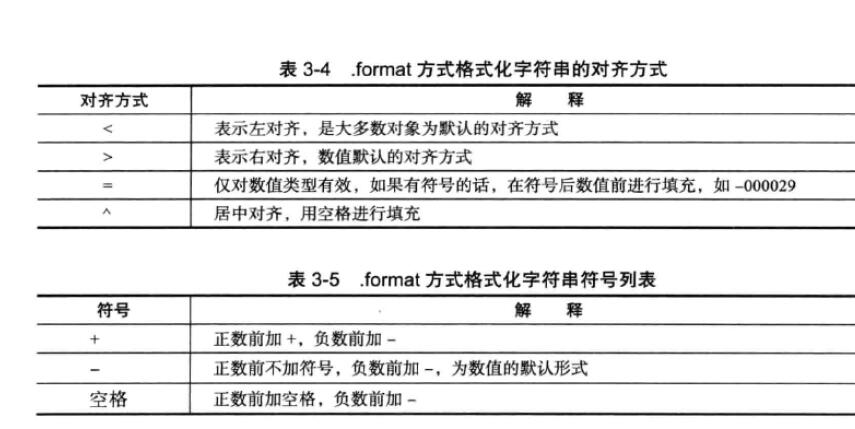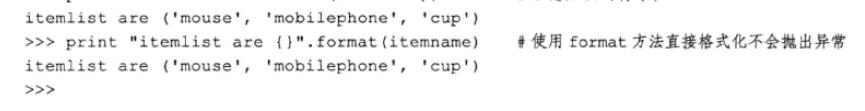# Python第二天  变量  运算符与表达式  input()与raw_input()区别  字符编码  python转义符  字符串格式化  format函数字符串格式化  帮助

Pycharm使用技巧（转载）

Python第一天  安装  shell  文件

Python第二天  变量  运算符与表达式  input()与raw_input()区别  字符编码  python转义符  字符串格式化

Python第三天 序列  5种数据类型  数值  字符串  列表  元组  字典

Python第四天   流程控制   if else条件判断   for循环 while循环

Python第五天   文件访问    for循环访问文件    while循环访问文件   字符串的startswith函数和split函数

Python第六天   类型转换

Python第七天   函数  函数参数   函数变量   函数返回值  多类型传值    冗余参数   函数递归调用   匿名函数   内置函数   列表表达式/列表重写

Python第八天  模块   包   全局变量和内置变量__name__    Python path

Python第九天  面向对象  类定义   类的属性    类的方法    内部类   垃圾回收机制   类的继承 装饰器

Python第十天   print >> f,和fd.write()的区别    stdout的buffer  标准输入 标准输出  标准错误   重定向 输出流和输入流

Python第十一天    异常处理  glob模块和shlex模块    打开外部程序和subprocess模块  subprocess类  Pipe管道  operator模块   sorted函数   生成器  walk模块   hashlib模块

Python第十二天     收集主机信息     正则表达式  无名分组   有名分组

Python第十三天   django 1.6   导入模板   定义数据模型   访问数据库   GET和POST方法    SimpleCMDB项目   urllib模块   urllib2模块  httplib模块  django和web服务器整合  wsgi模块   gunicorn模块

Python第十四天 序列化  pickle模块  cPickle模块  JSON模块  API的两种格式

Python变量

- 变量名由字母、数字、下划线组成。
- 变量不能以数字开头
- 不可以使用关键字
- a a1 _a

- 是变量的声明和定义的过程，等号两边可以有空格，而bash等号两边不能有空格
a = 1
id(a)

aa是整型
>>> aa=3
>>> aa
3

abc = ccc 报错 NameError: name 'ccc' is not defined
ab = 'ddd' 正确
>>> ab = 'ddd'
>>> print ab
ddd

Python运算符包括
- 赋值运算符
=：x = 3, y = ‘abcd’
+=： x += 2
-=：x -= 2
*=：x *= 2
/=： x /= 2
%=：x %= 2

- 算术运算符
+
-
*
/   除法，有小数
//  整除，没有小数
%
**   乘方 2**3=8 表示2的3次方

- 关系运算符
> ：1 > 2
< ：2 < 3
>=：1 >= 1
<=：2 <= 2
==：2 == 2
!=： 1 != 2

- 逻辑运算符
and逻辑与： True and False
or逻辑或： False or True
not逻辑非： not True

input()与raw_input()区别

input()与raw_input()返回值都是字符串

input() 输入的内容不能是字符，否则会当成变量
raw_input()   输入的内容不受限制

#!/usr/bin/python
#encoding:utf8
num1 = input("请输入数字: ")
num2 = input("Please input a number: ")

print "%s + %s = %s" % (num1, num2, num1+num2)
print "%s - %s = %s" % (num1, num2, num1-num2)
print "%s * %s = %s" % (num1, num2, num1*num2)
print "%s / %s = %s" % (num1, num2, num1/num2)
print "%s " % num1

print "%.2f" % (int(free)/1024.0)+'M'

format函数是Python 2.6 和Python 3.0 新增加的技术，是Python独有的方法，并且和%表达式的功能有不少的重叠。虽然%表达式目前还广泛使用

%表达式
%表示格式化字符串的格式符号。
%s，格式化字符串，字符，如果只有一个字符，通常不用括号，格式化之后变为字符串
%d，表示整数的字符串，格式化字符串，数字，如果只有一个字符，通常不用括号，格式化之后变为字符串
%x，表示16进制的字符串
%f，表示浮点数字符串，%.2f ，.2表示显示几位小数，如果只有一个字符，通常不用括号，格式化之后变为字符串，所以可以用+号连接'M'
%r，表示用rper()方法处理对象，打印时能够重现它所代表的对象(rper() unambiguously recreate the object it represents)，https://www.jb51.net/article/108589.htm

示例
import sys
i =10
sys.stdout.write("str:%d" %i)

import sys
i ='10'
sys.stdout.write("str:%s" %i)

import sys
i ='10'
print "str:%(key)s" % {'key':i}

import sys
i =10
sys.stdout.write("str:%02f" %i)

format()函数

print ('6:\t|{0:b}'.format(3))
print ('7:\t|{0:c}'.format(3))
print ('8:\t|{0:d}'.format(3))
print ('9:\t|{0:o}'.format(3))
print ('10:\t|{0:x}'.format(3))
print ('11:\t|{0:e}'.format(3.75))
print ('12:\t|{0:g}'.format(3.75))
print ('13:\t|{0:n}'.format(3.75))#浮点数
print ('14:\t|{0:n}'.format(3))  #整数
print ('15:\t|{0:%}'.format(3.75))#属性赋值，比较少用

class Customer(object):
def __init__(self,name):
self.name=name
def __str__(self):
return 'name : {self.name}'.format(self=self)

if __name__=='__main__':
print str(Customer('aa'))

#使用'{}'占位符
print('I\'m {},{}'.format('Hongten','Welcome to my space!'))
print('#' * 40)

#也可以使用'{0}','{1}'形式的占位符，位置符号
print('{0},I\'m {1},my E-mail is {2}'.format('Hello','Hongten','hongtenzone@foxmail.com'))
#可以改变占位符的位置
print('{1},I\'m {0},my E-mail is {2}'.format('Hongten','Hello','hongtenzone@foxmail.com'))
print('#' * 40)

#使用'{name}'形式的占位符，使用名称符号
print('Hi,{name},{message}'.format(name = 'Tom',message = 'How old are you?'))
print('#' * 40)

#混合使用'{0}','{name}'形式
print('{0},I\'m {1},{message}'.format('Hello','Hongten',message = 'This is a test message!'))
print('#' * 40)

 point = (1,2)
print '{0};{0}'.format(point)#下面进行格式控制
import math
print('The value of PI is approximately {}.'.format(math.pi))
print('The value of PI is approximately {!r}.'.format(math.pi))
print('The value of PI is approximately {0:.3f}.'.format(math.pi))

python字符串中的KeyError
'${ ss {} }'.formate('nihao') 报错 {}'''.format(HOST,PORT,USER,PASSWORD,timestampfile,timestampdoc,os.path.join(BACKDIR, now)) KeyError: '$and'

Python 会自动将format 函数的参数依次传递给{}占位符
print 'port={}'.format('11') + '\n'

print 'port={1},{1}'.format('11','22') + '\n'

print 'port={good}, haha is {bad}'.format(**d) + '\n'

format函数精度，符号，宽度，对齐方式，字符填充，逗号分隔进行测试
"{:.2f }".format(3.1415926)
'3.14'
"{:+.2f}".format(3.1415926)
'+3.14'
"{:10.2f}".format(3.1415926)
'3.14'
"{:^l0.2f}".format(3.1415926)
' 3.14  '
"{:,}".format(1234567)
'1,234,567'

https://segmentfault.com/q/1010000000334606

a='port=%s' + '\n' %(mongoport,)
TypeError: not all arguments converted during string formatting

print ('port=%d' + '\n') % (mongoport)
TypeError: %d format: a number is required, not str

python转义符

python里面有两种转义符

1、字符串格式化里面的转义符

2、正则表达式里面的转义符

1、%，两个特殊符号前面加%转义（字符串格式化里面的转义符）

sys.stdout.write("str:%d%%" %i)

"str:%d%%" %i ：百分之多少  %d  %  比如100%

sql = "grant replication slave on *.* to %s@'%%' identified by '%s'" %

%转义

2、\ 转义符  （正则表达式里面的转义符）

http://www.cnblogs.com/MYSQLZOUQI/p/5189884.html

python元字符：    .、^、$、*、+、?、{}、[]、\ 转义、|、() 我们想让通配符，或者元字符变成普通字符。那么这里我们就需要用到转义符了。 shell提供转义符有三种。 '' 单引号，硬转义，其内部所有的shell元字符、通配符都会被关掉。注意，硬转义中不允许出现’(单引号)。 "" 双引号，软转义，其内部只允许出现特定的shell元字符($,,\)：\$用于变量值替换、用于命令替换、\用于转义单个字符
\ 反斜杠，转义，去除其后紧跟的元字符或通配符的特殊意义。

http://www.2cto.com/os/201410/344020.html

"change master to master_host='%s'，master_port" %(abc)

intro = "He's a teacher"
statement = 'John said to me: "Can you do me a favour "'

intro = 'He\'s a teacher'
statement = "John said to me: \"Can you do me a favour \""

intro = r"He's a \ teacher"

""" type " license " for more information. Details about 'ob '， use ' object? for extra details . """

#encoding:utf8：指定字符编码支持中文

#encoding:utf8
#encoding:utf-8
#-*- encoding:utf-8 -*-
#coding:utf8
#coding:utf-8
#-*- coding:utf-8 -*-

dir()函数：看一下对象的属性，有哪些函数
help()函数
type()函数
ctrl+鼠标左键看源码，比如os.path，点进去会到__builtin__.py，不需要help函数这麽麻烦

type函数

type(变量名)

help函数

help(名称)

posted @ 2017-01-13 17:35  桦仔  阅读(...)  评论(... 编辑 收藏